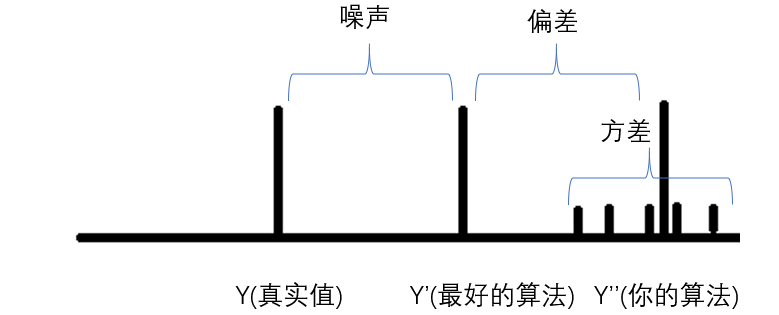## 机器学习导论-2 模型评估与选择

### 空间

• 假设空间

• 假设满足XX条件的是好瓜
• 版本空间

• 有限训练集，已知XX是好瓜
• 归纳偏好

• 假设空间和训练集一致的假设
• 学习过程中对某种类型假设的偏好称为归纳偏好
• No Free Lunch
• 奥卡姆剃刀：两个模型效果同样好，选择较为简单的

### 模型评估与选择

• 经验误差与过拟合

• 错误率率&误差
• 错误率：错份样本的占$E=a/m$
• 误差：样本真实输出与预测输出之间的差异
• 训练（经验）误差：训练集上
• 测试误差：测试集
• 泛化误差：初训练集外所有样本
• 过拟合

• 学习器把训练样本学习的“太好”，将训练样本本身的特点当作所有样本的一般性质，导致泛化性能下降
• 优化目标加正则项
• Early stop
• 欠拟合

• 对训练样本的一般性质尚未学好
• 决策树：扩展分支
• 神经网络：增加训练层数
• 评估方法

• 留出法
• 直接将数据集划分为两个互斥集合
• 训练/测试集划分要尽可能保持数据分布的一致性
• 一般若干次随机划分，重复实验取平均值
• 训练/测试样本比例通常为2:1～4:1
• 交叉验证法
• 将数据集分层采样划分为$k$个大小相似的互斥子集
• 自助法
• 以自助采样法为基础，对数据集$D$有放回采样$m$次得到训练集$D^{\prime}$，$D\backslash D^{\prime}$用作测试集
• 性能度量

• 性能度量是衡量模型泛化能力的评价标准，反映任务的需求

• 回归任务最常用的是“均方误差”：
• $E(f:D)=\frac{1}{m}\sum_{i=1}^{m}(f(x_i)-y_i)^{2}$
• 查准率 $P=\frac{TP}{TP+FP}$

• 查全率 $R=\frac{TP}{TP+FN}$

• $P-R$曲线：根据学习器的预测结果对样例排序，“最可能”的正例的在前面，排在最后的是“最不可能”是正例的样本，按此顺序把样本作为整理进行预测，每一次计算出当前的P,R然后以P为纵轴，R为横轴作图

• 如何利用多次训练得到了多个混淆矩阵？

• macro-F1:

也就是平均P,R之后再算

• micro-F1:

• $F1$ measure：$\frac{2\times TP}{N+TP-TN}$

• AUC预测了排序质量,越高越好；

AUC与排序的密切关系:考虑每一个正反例，若正例的预测值小于反例，那么就计算一个“罚分”：

• 代价敏感错误率:

损失是不一样大的一些任务里面，比如漏掉一个病人，放进去一个小偷等等，要考虑“非均等代价”

建立一个二分类代价矩阵里面有预测类别的cost,然后就就可以得到加权的代价敏感的错误率

这部分的重点是用户到底想要什么？标准型是什么？优化目标是什么？

• 性能评估

• 关于性能比较:某种度量取得评估结果之后能不能直接评价优劣？

• 测试性能并不等于泛化性能
• 测试性能随着测试集的变化而变化
• 很多机器学习算法本身有一定的随机性
• 直接选取相应评估方式在相应条件下评估并不可靠
• 二项检验

• 泛化错误率为$\epsilon$，测试错误率为$\hat{\epsilon}$，嘉定测试样本从样本总体分布中独立采样而来，我们可以使用“二项检验”，对于$\epsilon<epsilon_{0}$进行假设检验。
• 假设$\epsilon\leq\epsilon_{0}$，若测试错误率小于
• $t$检验

统计学的原来的方法：t检验中训练的数据可能不是那么独立，也许是一个“过高”的估计

• 交叉验证$t$检验

5*2交叉验证的含义：

两折：把数据分成两部分，一部分训练一部分测验；

五次：把数据洗五次，每次做两折；

目的：减小某一个数据集的误导

• McNemar检验:

关注正确-正确，错误-错误

• 多学习器比较:

使用Friedman+Nemenyi

算法的好坏不具有传递性：算法A比算法B好；算法B比算法C好；不等于A比C好

• 偏差和方差

• 对于测试样本$x$，令$y_{D}$为$x$在数据集中的标记，$y$为$x$的真实标记，$f(x;D)$为训练集$D$上学的模型$f$在$x$上的预测输出。

• 以回归任务为例：

• 期望预期为：$\bar{f}(x)=\mathbb{E}_{D}[f(x;D)]$；

• 使用样本数目相同的不同训练集产生的方差为$var(x)=\mathbb{E}_{D}[(f(x:D)-\bar{f}(x))^{2}]$；(每次做的浮动范围)

• 噪声为$\varepsilon^{2}=\mathbb{E}_{D}[(y_{D}-y)^{2}]$: 本真误差

• 偏差$bias^2(x)=(\overline{f}(x)-y)^2$ 期望输出和真实输出的差别

比较清晰的图示(周老师真nb):• $E(f;D)=bias^{2}(x)+var(x)+\varepsilon^2$

一开始偏差(bias)起到主要的作用，后来是数据造成的扰动(方差)起到主要的作用，然后overfitting一般情况下是在后者气主要作用的时候产生的，因为其实学习的是数据本身的特征